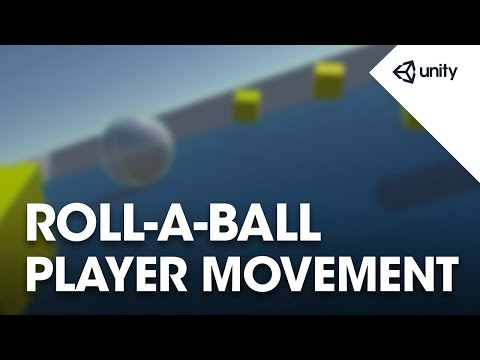# Moving the Player

#### Сложность: Базовая

Moving the player object using player input and physics forces.## Moving the Player

Базовая Roll-a-ball tutorial

This is the current state of this script, as of the end of this lesson. This script will continue to change and mature in later lessons.

### Code snippet

``````using UnityEngine;
using System.Collections;

public class PlayerController : MonoBehaviour {

public float speed;

private Rigidbody rb;

void Start ()
{
rb = GetComponent<Rigidbody>();
}

void FixedUpdate ()
{
float moveHorizontal = Input.GetAxis ("Horizontal");
float moveVertical = Input.GetAxis ("Vertical");

Vector3 movement = new Vector3 (moveHorizontal, 0.0f, moveVertical);

rb.AddForce (movement * speed);
}
}``````

#### Связанные обучающие материалы#### Roll-a-ball tutorial

1. Setting up the Game
2. Moving the Player